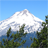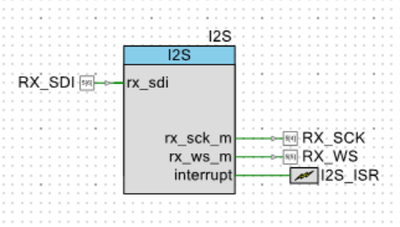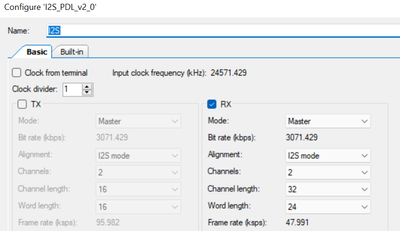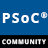# Reading clock frequency value in runtimeLevel 4
Level 4Hi

I need to get the Frame rate and send over the UART for user-feedback.

I think I need to start with the I2S clock frequency or Bit rate during then calculate the frame rate.

Question 1: how can I read the  I2S clock frequency or Bit rate ? in other words, which variable is associated with these values so that I can read ?

Question 2: If I am using an external clock source, for example Clk_Peri, how can I read its value ?

thanks1 Solution

# Re: Reading clock frequency value in runtimeEmployee
EmployeeAnswering question (2) first. You can't use Clk_Peri directly to the I2S block. The I2S block only accepts two sources:

- HFCLK1, which you can configure in the Design Wide Resources

- External clock to i2s_if pin (P5_0)

Answering your question (1), if you are using HFCLK1, you can use the function Cy_SysClk_ClkHfGetFrequency(1) to get the frequency. If you are using the external clock, you need to know the frequency.

To calculate the bit rate, use this formula:

BIT_RATE = M_FREQ / I2S_CLOCK_DIV / 8

Where M_FREQ is the frequency you are driving the I2S block (HFCLK1 or i2s_if). I2S_CLOCK_DIV is the divider set in the I2S component.

To calculate the frame rate, use this formula:

FRAME_RATE = BIT_RATE / CHANNEL_LENGTH / NUM_CHANNELS

2 Replies

# Re: Reading clock frequency value in runtimeEmployee
EmployeeAnswering question (2) first. You can't use Clk_Peri directly to the I2S block. The I2S block only accepts two sources:

- HFCLK1, which you can configure in the Design Wide Resources

- External clock to i2s_if pin (P5_0)

Answering your question (1), if you are using HFCLK1, you can use the function Cy_SysClk_ClkHfGetFrequency(1) to get the frequency. If you are using the external clock, you need to know the frequency.

To calculate the bit rate, use this formula:

BIT_RATE = M_FREQ / I2S_CLOCK_DIV / 8

Where M_FREQ is the frequency you are driving the I2S block (HFCLK1 or i2s_if). I2S_CLOCK_DIV is the divider set in the I2S component.

To calculate the frame rate, use this formula:

FRAME_RATE = BIT_RATE / CHANNEL_LENGTH / NUM_CHANNELS

# Re: Reading clock frequency value in runtimeLevel 4
Level 4Hi Rodolf, thanks a lot, will try it.